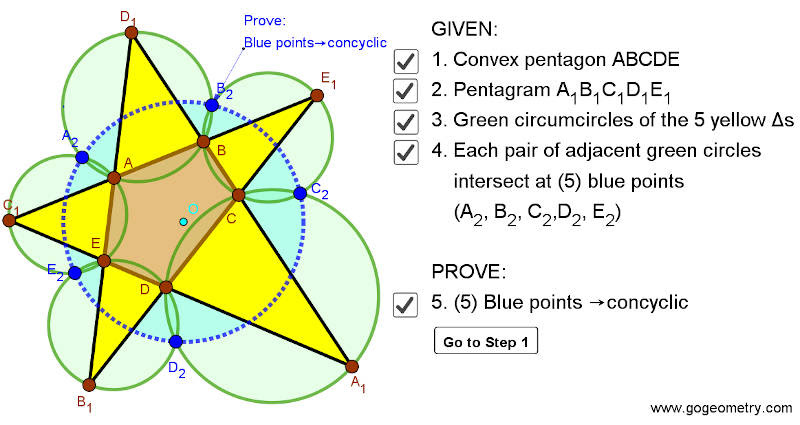## Friday, May 1, 2020

### Dynamic Geometry 1477: Miquel's Pentagram Theorem, Pentagon, Triangle, Circumcircles, Concyclic Points, Step-by-step Illustration

Interactive step-by-step animation using GeoGebra. Post your solution in the comment box below.
Level: Mathematics Education, High School, Honors Geometry, College.

Details: Click on the figure below.1.Consider Triangle A1ED1, B1DC and C1AB are transversals.
Points D2 and A2 will also lie on circumcircle of triangle A1ED1.
Angle EA2D2= Angle EA1D2= Angle DC2D2= x,
Let Angle E2A2E=y. Angle E2A2D2=Angle EA2D2+Angle EA2E2=x+y

Now consider triangle E1C1D, AEB1 and BCA1 are transversal.
Point C2 and E2 lie on circumcircle of triangle E1C1D.
Angle E2C2D = Angle E2C1D = Angle E2A2E = y
Angle E2C2D2= Angle E2C2D + Angle DC2D2= x+y
We have Angle E2A2D2 = Angle E2C2D2, hence A2,C2,D2,E2 are concyclic.
Similarly B2 can be proven concyclic.

2.https://photos.app.goo.gl/QCqGuc6qFkjEkbWG7

I would like to add some clarification to his solution.
Consider circumcircles of triangles BDD1 and CDA1 and 2 secants BDA1 and CDB1 . these secants meet at D1
Per the result of problem 948 ( see link above)
A1, B1, D1 and D2 are cocyclic ( see blue circle)
Similar result to circumcircles of triangles AEC1 and ABD1 and 2 secants BAC1 and EAD1 we will have A2 on blue circles

1.Thanks Peter for your kind words.
Also thanks for sharing nice diagram, it provides more clarity.

Regards